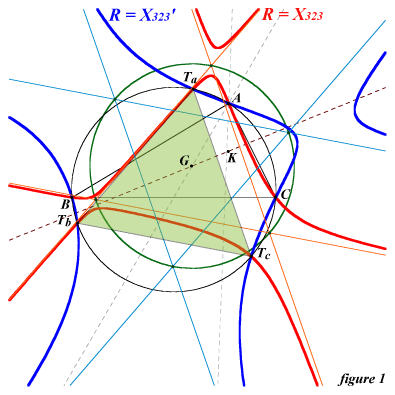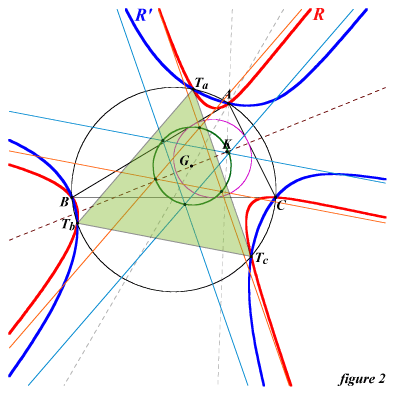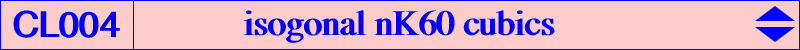All isogonal nK60 cubics have their root R on the line GK. They meet the circumcircle at A, B, C and three other points Ta, Tb, Tc which are the isogonal conjugates of the points at infinity of the sidelines of the Morley triangle. these points are the vertices of the circumtangential triangle. They have three real asymptotes parallel to the sidelines of this Morley triangle. Thus, they form a pencil of cubics generated by two special cases : when the root is G, the cubic decomposes into the line at infinity and the circumcircle when the root is K, the cubic is the only nK0 and nK+ of the pencil : it is the Kjp cubic K024. If R is defined by vector(GR)= t vector(GK), the barycentric equation of the pencil is :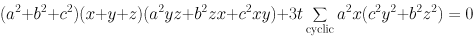The triangle formed by the asymptotes is always centered at G (except of course the case R = G) and its circumradius is :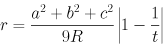If R is different of G and K, there is another point R' such that the two corresponding triangles have the same circumradius r : R' is the harmonic conjugate of R with respect to G and K. In figure 1, R = X(323) and r is the radius of the circumcircle of ABC. In figure 2, R and R' are not mentioned in the current edition of Clark Kimberling's ETC. Their barycentric coordinates are : 2 a^2(a+b+c) ± 3 abc : : . In both cases, r is the radius of the incircle. Other examples of such cubics are K085, K098, K105, K686.